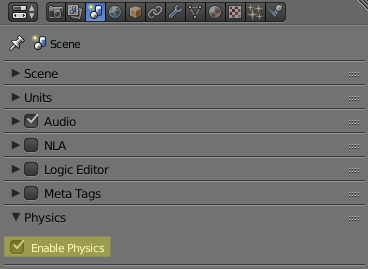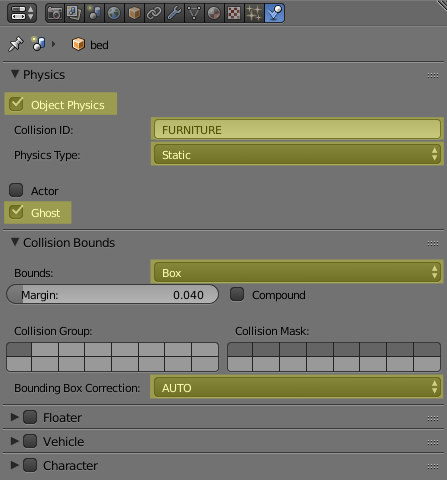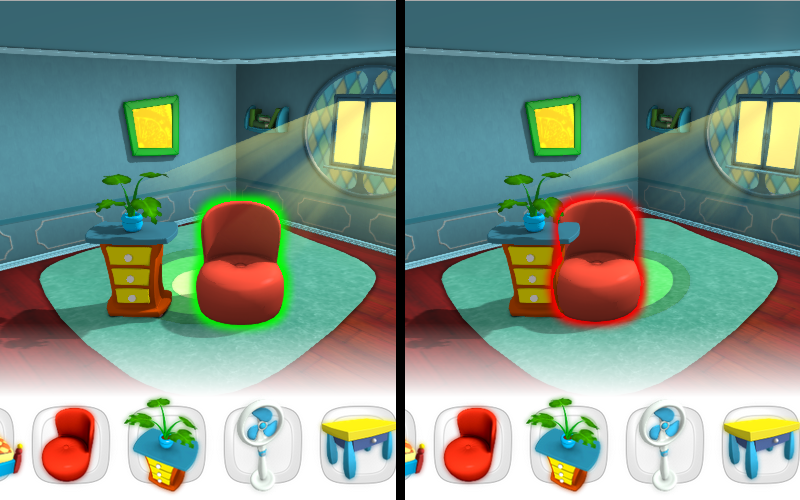# Furnishing a Room Part 2: Interactivity and Physics

2014-08-22

Now we'll continue with our "Playroom" app. In the previous article of the series we looked at the dynamic loading technology. In this one we'll familiarize ourselves with such important app components as the physics and control system.

### Setting Up Physics

Remember we prepared a bunch of scene files: the main scene with the room and additional ones with the furniture items.

The furniture items will physically interact with each other but in a simple way - there will be a notification when they collide with similar objects. For this we'll enable physics simulation for the main scene by activating the Enable Physics checkbox on the Scene > Physics panel.As for the additional scenes we need to set up the physics properties for every furniture item because we want to detect their collisions with each other. We can do that under the Physics tab.

On the Physics panel let's enable Object Physics and type an id for this object into the Collision ID text field. For all objects this value will be the same: FURNITURE.

For the object's Physics Type select the Static option. This seems counter-intuitive on the first glance because these objects will be moved by the user. Nevertheless in this case we'll need to choose exactly this physics interaction type - while collisions are detected for the objects, the latter themselves are not moved by the forces.

Then we enable the Ghost checkbox - this will allow to detect contacts but will not prevent collisions between objects.

For each object select the most appropriate bounding volume type Collision Bounds > Bounds. The following types are supported: Capsule, Box, Sphere, Cylinder and Cone. Also leave the default AUTO value for the Bounding Box Correction.In detail these and other physics settings are described in the corresponding section of the user manual.

### Interactivity: Rotations

In our "Playroom" app the user may arrange the furniture by rotating and moving the items inside the room.

The furniture object rotation occurs when the user clicks the corresponding buttons in the app interface:

```function init_controls() {
...
if (_selected_obj)
rotate_object(_selected_obj, ROT_ANGLE);
});
if (_selected_obj)
rotate_object(_selected_obj, -ROT_ANGLE);
});
...
}
```

This is implemented in the rotate_object() function. Inside it we used a quaternion, which we rotated by the angle around the vertical axis and assigned to the object again:

```function rotate_object(obj, angle) {
var obj_parent = m_obj.get_parent(obj);

if (obj_parent && m_obj.is_armature(obj_parent)) {
// rotate the parent (armature) of the animated object
var obj_quat = m_trans.get_rotation(obj_parent, _vec4_tmp);
m_quat.rotateY(obj_quat, angle, obj_quat);
m_trans.set_rotation_v(obj_parent, obj_quat);
} else {
var obj_quat = m_trans.get_rotation(obj, _vec4_tmp);
m_quat.rotateY(obj_quat, angle, obj_quat);
m_trans.set_rotation_v(obj, obj_quat);
}
limit_object_position(obj);
}
```

### Interactivity: Moving

The user may drag the furniture items with the mouse or with a touch.

We'll start by registering the necessary event listeners:

```function init_cb(canvas_elem, success) {
...

...
}
```

Upon clicking the main_canvas_down() callback is executed. Here we'll get the screen coordinates of the clicking point and pick the object:

```function main_canvas_down(e) {
...

var x = m_mouse.get_coords_x(e);
var y = m_mouse.get_coords_y(e);

var obj = m_scenes.pick_object(x, y);

...

// calculate delta in viewport coordinates
if (_selected_obj) {
var cam = m_scenes.get_active_camera();

var obj_parent = m_obj.get_parent(_selected_obj);
if (obj_parent && m_obj.is_armature(obj_parent))
// get translation from the parent (armature) of the animated object
m_trans.get_translation(obj_parent, _vec3_tmp);
else
m_trans.get_translation(_selected_obj, _vec3_tmp);
m_cam.project_point(cam, _vec3_tmp, _obj_delta_xy);

_obj_delta_xy = x - _obj_delta_xy;
_obj_delta_xy = y - _obj_delta_xy;
}
}
```

When the user tries to move the furniture the main_canvas_move() function is called in which the objects will follow the cursor. To use the controls more conveniently we'll turn the camera controls off in this moment:

```function main_canvas_move(e) {
if (_drag_mode)
if (_selected_obj) {
// disable camera controls while moving the object
if (_enable_camera_controls) {
m_app.disable_camera_controls();
_enable_camera_controls = false;
}

// calculate viewport coordinates
var cam = m_scenes.get_active_camera();

var x = m_mouse.get_coords_x(e);
var y = m_mouse.get_coords_y(e);

if (x >= 0 && y >= 0) {
x -= _obj_delta_xy;
y -= _obj_delta_xy;

// emit ray from the camera
var pline = m_cam.calc_ray(cam, x, y, _pline_tmp);
var camera_ray = m_math.get_pline_directional_vec(pline, _vec3_tmp);

// calculate ray/floor_plane intersection point
var cam_trans = m_trans.get_translation(cam, _vec3_tmp2);
m_math.set_pline_initial_point(_pline_tmp, cam_trans);
m_math.set_pline_directional_vec(_pline_tmp, camera_ray);
var point = m_math.line_plane_intersect(FLOOR_PLANE_NORMAL, 0,
_pline_tmp, _vec3_tmp3);

// do not process the parallel case and intersections behind the camera
if (point && camera_ray < 0) {
var obj_parent = m_obj.get_parent(_selected_obj);
if (obj_parent && m_obj.is_armature(obj_parent))
// translate the parent (armature) of the animated object
m_trans.set_translation_v(obj_parent, point);
else
m_trans.set_translation_v(_selected_obj, point);
limit_object_position(_selected_obj);
}
}
}
}
```

In order to detect the future object position we'll get the coordinates (in the screen space) of the point to which the object center will be projected after it is moved. Then we build a three-dimensional vector directed from the camera position to this point, and find out the intersection of this vector with the room floor plane. This intersection point will be our position we looked for. Then just place the object there.

Now let's look at line_plane_intersect() in more detail:

```var cam_trans = m_trans.get_translation(cam, _vec3_tmp2);
m_math.set_pline_initial_point(_pline_tmp, cam_trans);
m_math.set_pline_directional_vec(_pline_tmp, camera_ray);

var point = m_math.line_plane_intersect(FLOOR_PLANE_NORMAL, 0,
_pline_tmp, _vec3_tmp3);
```

It serves for finding out the point of the line-plane intersection. The first two arguments define the plane - namely its normal (FLOOR_PLANE_NORMAL) and the distance (which is zero) from this plane to the origin of coordinates. These values fit the room model. Also we pass a special object (_pline_tmp) - a line in a 3D space that represents the ray emitted from the camera's position along its view vector.

Finally main_canvas_up() restores camera controls when the movement has finished:

```function main_canvas_up(e) {
...
if (!_enable_camera_controls) {
m_app.enable_camera_controls();
_enable_camera_controls = true;
}
...
}
```

### Wall Limitations

The furniture objects are moved in such a way that they are always parallel to the room floor. To avoid going beyond the room walls we use the limit_object_position() function:

```function limit_object_position(obj) {
var bb = m_trans.get_object_bounding_box(obj);

var obj_parent = m_obj.get_parent(obj);
if (obj_parent && m_obj.is_armature(obj_parent))
// get translation from the parent (armature) of the animated object
var obj_pos = m_trans.get_translation(obj_parent, _vec3_tmp);
else
var obj_pos = m_trans.get_translation(obj, _vec3_tmp);

if (bb.max_x > WALL_X_MAX)
obj_pos -= bb.max_x - WALL_X_MAX;
else if (bb.min_x < WALL_X_MIN)
obj_pos += WALL_X_MIN - bb.min_x;

if (bb.max_z > WALL_Z_MAX)
obj_pos -= bb.max_z - WALL_Z_MAX;
else if (bb.min_z < WALL_Z_MIN)
obj_pos += WALL_Z_MIN - bb.min_z;

if (obj_parent && m_obj.is_armature(obj_parent))
// translate the parent (armature) of the animated object
m_trans.set_translation_v(obj_parent, obj_pos);
else
m_trans.set_translation_v(obj, obj_pos);
}
```

Now I'll shortly explain what's going on inside it. First, we know the wall coordinates because we know how the room model looks like. In the script they are defined as constants WALL_X_MAX, WALL_X_MIN, WALL_Z_MAX and WALL_Z_MIN. Second, the furniture objects (like all other objects) have their bounding box coordinates. This altogether allows us to detect when the objects are coming out the room and correct their positions.

### Collision Detection

For clearness and convenience we'll outline the colliding furniture:This is implemented using sensors.

After each scene is dynamically loaded, loaded_cb(), which was passed into data.load() as an argument, is called:

```function init_controls() {
...
});
...
}

var objs = m_scenes.get_all_objects("ALL", data_id);
for (var i = 0; i < objs.length; i++) {
var obj = objs[i];

if (m_phy.has_physics(obj)) {
m_phy.enable_simulation(obj);

// create sensors to detect collisions
var sensor_col = m_ctl.create_collision_sensor(obj, "FURNITURE");
var sensor_sel = m_ctl.create_selection_sensor(obj, true);

if (obj == _selected_obj)
m_ctl.set_custom_sensor(sensor_sel, 1);

m_ctl.create_sensor_manifold(obj, "COLLISION", m_ctl.CT_CONTINUOUS,
[sensor_col, sensor_sel], logic_func, trigger_outline);

...
}

...
}
}
```

Here we get newly loaded objects on the scene:

```var objs = m_scenes.get_all_objects("ALL", data_id);
```

...then for each object we check if it is physical:

```...
if (m_phy.has_physics(obj)) {
...
}
...
```

...and for the physical objects create a pair of sensors:

```var sensor_col = m_ctl.create_collision_sensor(obj, "FURNITURE");
var sensor_sel = m_ctl.create_selection_sensor(obj, true);
```

The create_collision_sensor() method creates a sensor for detecting intersections with other physical objects. The obj object is passed as the first parameter. On this object the collision sensor is registered. The second argument - Collision ID - was assigned to the objects in Blender. Therefore this sensor will notify us when the obj object collides with any object with such a Collision ID.

In our example all the furniture items have the same FURNITURE id and so interact only with each other.

The create_selection_sensor() method creates a sensor to notify us when the object is selected.

Now create a container for the sensors - a so called sensor manifold:

```m_ctl.create_sensor_manifold(obj, "COLLISION", m_ctl.CT_CONTINUOUS,
[sensor_col, sensor_sel], logic_func, trigger_outline);
```

Now some explanations:

• obj - the object on which the sensor manifold is registered;
• "COLLISION" - the collision manifold id; it must be unique for each object on which the manifoild is registered;
• CT_CONTINUOUS - the manifold type is selected so that the callback function would execute every time when the logic function returns True;
• sensors - sensor array constituting the manifold;
• logic_func - the logic function; its result together with the manifold type defines when and how often the callback is executed;
• trigger_outline - the callback function.

The array of all sensor values is passed to the logic_func as argument. In our example this function returns True for the selected object:

```function logic_func(s) {
return s;
}
```

The trigger_outline() function is quite simple:

```function trigger_outline(obj, id, pulse) {
if (pulse == 1) {
// change outline color according to the
// first manifold sensor (collision sensor) status
var has_collision = m_ctl.get_sensor_value(obj, id, 0);
if (has_collision)
m_scenes.set_outline_color(OUTLINE_COLOR_ERROR);
else
m_scenes.set_outline_color(OUTLINE_COLOR_VALID);
}
}
```

Here the pulse argument - generated by the sensor manifold - is used. The pulse depends on the logic function result and the sensor manifold type. Particularly the pulse will be positive for the CT_CONTINUOUS type (pulse = 1), if the logic function is true, and negative (pulse = -1), if it is false.

In case of positive pulse, which implies the selected object, we will determine the collisions. To achieve this we will check the state of the appropriate sensor:

```var has_collision = m_ctl.get_sensor_value(obj, id, 0);
```

The last parameter here defines the sensor's index in the manifold, which in our example has 2 sensors.

We set outline color to red (OUTLINE_COLOR_ERROR constant) for the selected object in case of collisions. If there are no collisions the selected object is outlined with green (OUTLINE_COLOR_VALID). In that way we clearly visualize collisions between furniture items.

### Conclusion

Today we have looked at the components of our app that are responsible for physics simulation and interactions with the user. Such details are very important because they contribute to interactivity and make the gaming process more lively and exciting.

Here we finish describing the coding part of our "Playroom" app. The next article in this series will be about doing the Blender modeling for this app.

The source files for this example are available as part of the Blend4Web SDK free distribution.

Run the application in a new window

### Changelog

[2014-08-22] Initial release.

[2014-10-29] Updated the example code because of API change.

[2014-12-23] Updated the example code because of API change.